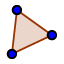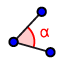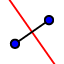# Geogebra_lesson_1

Author:
dlebair

## Getting started...

1.  Draw a triangle with vertices (-3, 4), (2,4), and (1,1).  Useat the top. 2.  Find the lengths of each side of the triangle by using the measurements on the left side.     BC =     AC =      AB =  Classify the triangle by its sides: _____________________ 3.  Measure the angles of the triangle, using.  Click in the order  so that the second side  you click is a clockwise rotation from the first.  All your angles should be acute.  If not, please re-measure. 4.  Find the incenter of the triangle by bisecting all three angles of the triangle..  What are the coordinates of the incenter?   ______________ 5.   Clear the page except for triangle ABC. 6.  Draw three perpendicular bisectors for the triangle, and find the circumcenter.What are the coordinates of the circumcenter? ______________ 7.  Translate the triangle 5 units right.  What are the coordinates? ___________________ 8.  Rotate the triangle 45 ccw about the point (2,1).  What are the new coordinates? _______________ 9.  Reflect A'B'C' over the y-axis.  What are the coordinates? 10.  Take a screen shot of your figures, and copy it to a word file.  Upload the word file with your name in this format to Canvas:       Deborah_Lebair_lesson_1